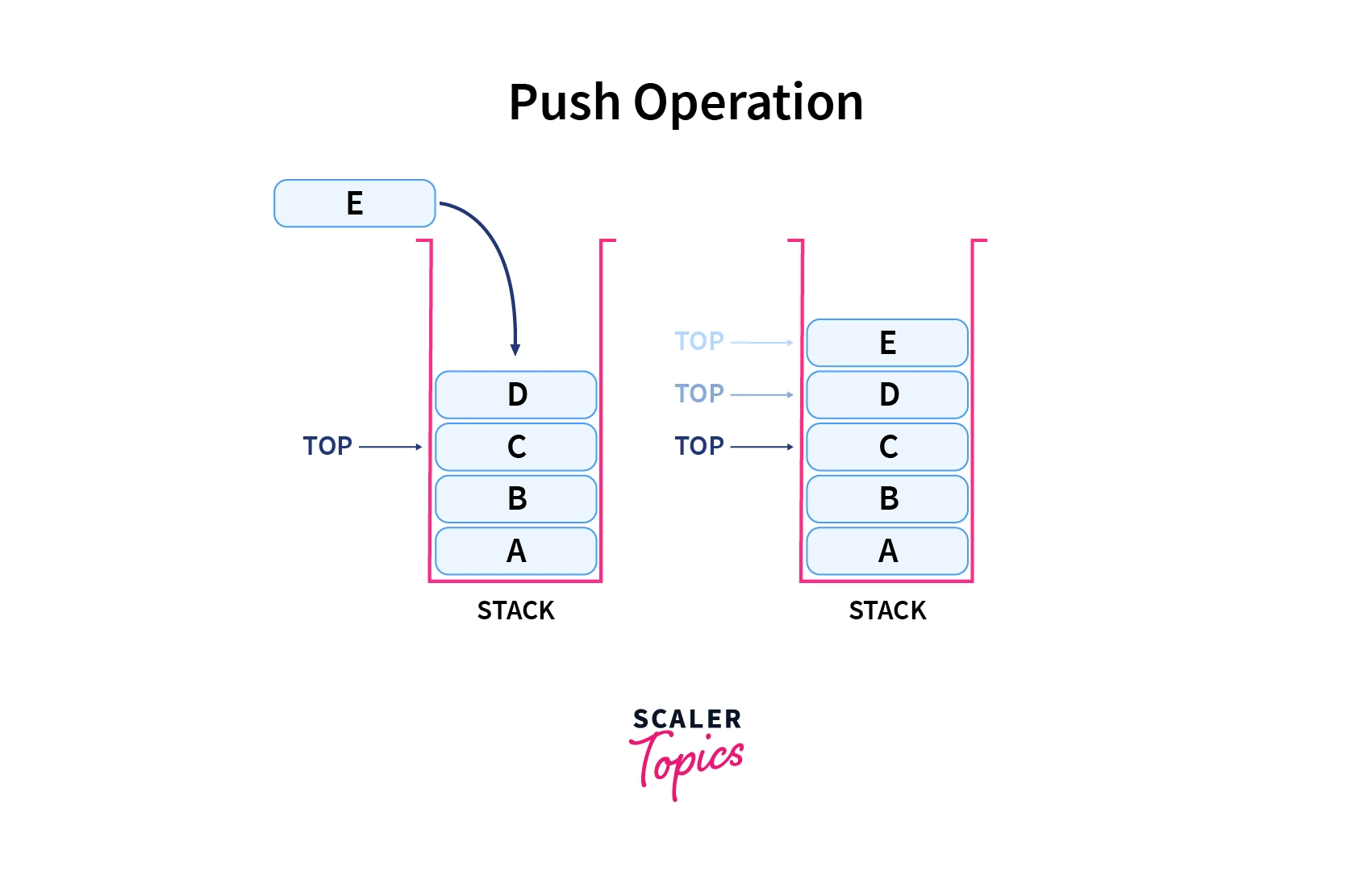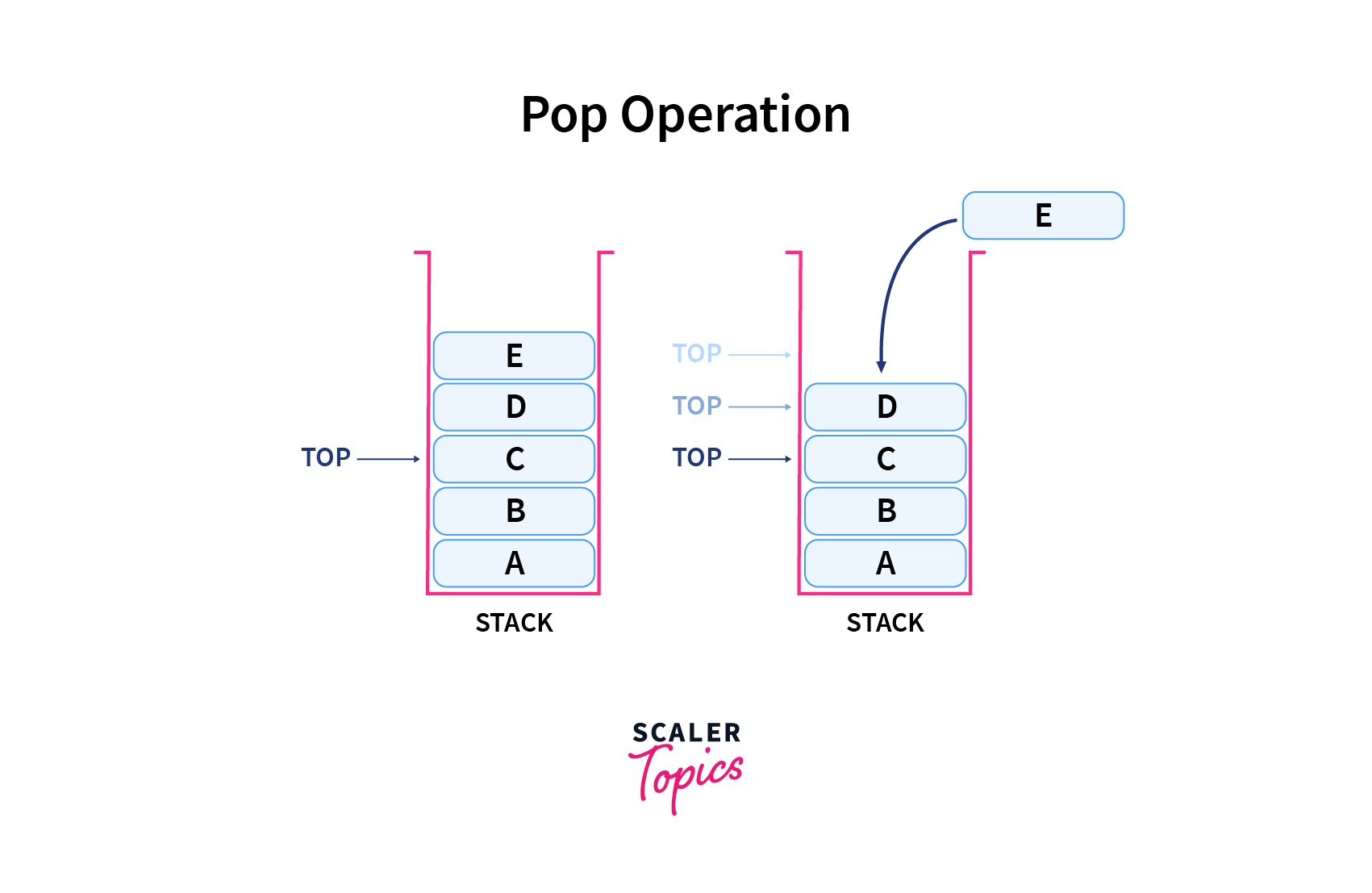# Stack in Python

Challenge Inside! : Find out where you stand! Try quiz, solve problems & win rewards!
Learn via video coursePython Course for Beginners With Certification: Mastering the Essentials
By Rahul JanghuFree
4.90Enrolled: 1000Python Course for Beginners With Certification: Mastering the Essentials
Rahul JanghuFree
4.90
Enrolled: 1000

## Overview

A stack is a linear data structure that stores items in a Last In First Out way. In stack, elements are added at one end and an element is deleted from that end only. The insert and delete operations are called push and pop operations.

As an example, the data in the stack is stored in a similar order as dish plates are stacked one above another on the dining table.

Stack in Python Programming can be implemented by using List, collections class deque, queue module, or linked-list.

## Introduction Stack in Python

Stack is a abstract data type that can be defined as a linear collection of data items that supports last in and first out (LIFO) semantics for insertion and deletion of data elements.

Talking about performance, a efficient stack implementation is expected to take order O(1) time complexity for insert and delete operations.

To understand Stack at the ground level, think about a pile of plates in a spring-board. You add a dinner plate at the top of the stack of plates, so the first one to be picked up will be the last one that was added to the stack of plate.

A stack in python is a linear data structure that stores items in a Last-In/First-Out manner i.e you put items in and then you get them out in the exact reverse order that you put them in. This is frequently referred to as LIFO. This opeartes in similar way to a queue, which stores items in a First-In and First-Out (FIFO) manner. In any stack structure, a new data object or element is added at one end and removed from the same end only. The insert and delete operations performed on stack are often termed as push (inserting an element) and pop(deleting an element).

Push operation as demonstrated in below diagram will add an element at the top of stack. Refer the below image for more understanding:Pop operation as demonstrated in below diagram removes an element from the top of the stack. Refer the below image for more understanding:## How to Create a Stack in Python?

We can create a stack in python using the built-in List data structure which comes with methods to simulate stack and queue operations. The stack can also be created using the deque library in python which efficiently provides stack and queue operations in one object. Lastly, a stack in python can also be created by using the LifoQueue class.

## Methods of Python Stack

The functions/methods associated with stacks are listed below:

• empty() – Returns a boolean (True/False) value as True if the stack is empty
• size() – Returns size of the stack or number of items/elements stored in the stack
• top() – Returns a reference to the topmost available element stored in the stack
• push(a) – Inserts an element at the top of the stack
• pop() – Deletes the topmost element from the last occupied memory location of the stack

## Implementation of Stack in Python

Stack in python can be implemented in various ways using existing forms of data structures. Python stack can be implemented using the following ways:

### Implementation Using List

Stack in Python can be implemented simply by using a list data structure. Stack function push() can be mimicked by using the append() function which adds the elements to the top of the stack.

Unfortunately, the list has a few shortcomings.

• The concerning issue is that this implementation of stack using the list can run into performance issues as it grows in terms of time complexity. The objects/elements in the list are stored next to each other in the block of memory.
• When Stack grows bigger, Python does some additional memory allocations on the block of memory that currently holds it. This can lead to some append() calls (to add items to the list) taking a much longer duration than other ones.

We can use the pop() method of the list data type to write the pop method that pops out the topmost element from the stack.

The list stores the new element in the next block to the last one. If the list grows out of a block of memory then Python allocates some memory. That's why the list becomes slow. Let's understand the following example:

In below mentioned Python program, the stack has been implemented in Python and the basic operational function assumes that the end of the list will hold the top element of the stack.

As the stack grows new items will be added at the end of the list. pop operations will manipulate the list and take out the items from the same end.

Output

### Implementation Using Collections Deque Methods

An alternate way to implement stack using Python coding is by using the deque class from the collections module.

The deque class in the Python library can be used to implement a double-ended queue which supports the addition push() and removal pop() of elements from either end in O(1) time complexity.

Deques support the addition and removal of elements from both ends of queue/stack equally well hence they can serve both as stacks and as queues. Deque collections class is also a preferred implementation over the list in the cases where we need fast append and pop operations from both the ends of the container.

This is possible only because deque provides an O(1) time complexity for append and pop operations as compared to a list with amortized O(1).

The below stack implementation in Python (attached below Program) uses the deque class from the collection module. Used append operation to add new items in stack and used pop() function to take out the items from the same end to follow LIFO sequence.

Output

### Implementation Using Queue Module

The queue module available in the Python library contains many classes used for implementing multi-producer and multi-consumer queues that we can use for parallel computing.

To implement a stack in python using a queue module you can use a list or a deque as a general-purpose stack.

The queue module has a Last-In-First-Out Queue feature, which is a Stack. Data or elements are inserted into Queue using the put() function (alternatively called as push) and get() takes data out from the Queue.

Various functions are available and can be used as per the explanation below:

• maxsize – Number of items/objects allowed to be stored in the queue.
• empty() – Return a boolean value as True if the queue is empty else False.
• full() – Return True if queue contains maxsize items. If queue gets initialized with maxsize=0, then full() never returns True.
• get() – This function removes and returns an item from the queue to the calling function. In case the queue is empty or NULL, the get() function will wait until a new element is available.
• get_nowait() – Return an item if item is immediately available, else raise QueueEmpty flag.
• put(item) – Put/Push an item/object into the queue. If the queue is full, the put() function will wait till the time a free memory slot is available before adding a new element.
• qsize() – Return the number of items/objects in the queue.

Python program below demonstrates stack implementation (attached below Program) uses queue module using Python library and operates basic stack operations using in-built functions of queue module like put('element'), full(), get(), etc.

Output

### Implementation Using Singly Linked List

To implement stack in Python, a linked list can be used and basic stack operations can be mimicked by methods addHead (item) and removeHead(). These methods get executed in constant time.

• getSize()– Return the total size of the stack i.e number of items/elements stored in the stack.
• peek() – Function would return the top item/element in the stack. In the case of an empty stack, the peek function would raise an exception alert.
• pop() – Function would take out (remove) and return a value of the last entered element of the stack. In the case of an empty stack, the pop() function would raise an exception alert.
• isEmpty() – Return a boolean value True if the stack is empty and false otherwise.
• push(value) – A new element/object is entered into the head of the stack.

Output

### Which Implementation of Stack Should We Consider?

Among all available techniques used to implement Stack in Python, you should use the deque method in case you’re not using threading.

If you are using threading, then an efficient way to implement a stack is LifoQueue unless the performance of push and pop operations is in a limited range.

The list may be a familiar and easy technique, but it should be avoided most of the time because it can potentially have memory reallocation issues that may impact performance. Finally, the interfaces for deque and list are identical, and deque doesn’t have these issues, which makes deque the best choice.

## Benefits of Stack in Python

• Stack in Python can allocate memory dynamically which cannot be done in the list. • list in Python takes a lot of effort to add a new element or remove an element whereas stack in Python can easily manage the addition or removal of elements.

## Summary

Now you know what is stack and have seen scenarios where stack can be used in real-life programs. By now, you must have learned the following:-

• Acquired a sound understanding of what a python stack is and how it can be implemented using different well-defined data structures and library functions used in Python
• How to implement different techniques to deploy stacks in python
• Observed that deque is a great choice for non-threaded programs
• In the case of threading environment, a good idea would be to use a LifoQueue

Now that we have clearly defined and demonstrated the implementation technique of stack in python, you can turn your attention to using Python to implement the stack.